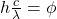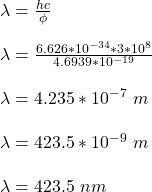## What is the maximum wavelength of incident light that can produce photoelectrons from silver? The work function for silver is Φ=2.93 eV.

Question

What is the maximum wavelength of incident light that can produce photoelectrons from silver? The work function for silver is Φ=2.93 eV.

in progress 0
1 month 2021-08-07T20:31:09+00:00 1 Answers 6 views 0

The maximum wavelength of incident light that can produce photoelectrons from silver is 423.5 nm.

Explanation:

Given;

work function of silver, Φ = 2.93 eV = 2.93 x 1.602 x 10⁻¹⁹ J = 4.6939 x 10⁻¹⁹ J

Apply Einstein Photo electric effect;

E = K.E + Ф

Where;

E is the energy of the incident light

K.E is the kinetic of electron

Ф is the work function of silver surface

For the incident light to have maximum wavelength, the kinetic energy of the electron will be zero.

E = Ф

hf = Фwhere;

c is speed of light = 3 x 10⁸ m/s

h is Planck’s constant, = 6.626 x 10⁻³⁴ J/s

λ is the wavelength of the incident lightTherefore, the maximum wavelength of incident light that can produce photoelectrons from silver is 423.5 nm.